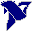# LabVIEW

cancel
Showing results for
Search instead for
Did you mean:

## Reuleaux triangle

Dear forum experts,

I have to draw a Reuleaux triangle (non-obvious constant width curve) using Labview, like the one in the attached somatochart file.

The program will be used in an anthropometry laboratory to indentify the somatotype (endomorph, ectomorph or mesomorph) of individuals.

Can you give mer any suggestions?

Thanks,

Davide

Message 1 of 6
(1,331 Views)

## Re: Reuleaux triangle

Hi Davide,

use a XY graph and draw your curve.

What exactly is the problem?

Best regards,
GerdWusing LV2011SP1 + LV2017 (+LV2020 sometimes) on Win10+cRIO
Message 2 of 6
(1,312 Views)

## Re: Reuleaux triangle

Dear Gerd,

I understand I am missing something... but the triangle is an area, not a function. The total area is 0.5(pi-sqrt(3))R^2.

R is the constant radius of the three intersecting circles.

Actually, I have no clue where to begin from...

Thanks,

Davide

Message 3 of 6
(1,303 Views)

## Re: Reuleaux triangle

Hi Davide,

you need to know the inner triangle. Once you know its corner points you can draw arcs (or better: calculate XY points for a plot). It looks like simple geometry math!

Now I looked at Wikipedia and I can also suggest to use a 2d picture indicator for your Releaux triangle plot. Here you could use draw functions directly instead of calculating points in a XY graph…

Best regards,
GerdWusing LV2011SP1 + LV2017 (+LV2020 sometimes) on Win10+cRIO
Message 4 of 6
(1,296 Views)

## Re: Reuleaux triangle

Thanks Gerd,

the inner triangle is equilateral, the side being R.

What is a 2d picture indicator?

Thanks

Davide

Message 5 of 6
(1,291 Views)

## Re: Reuleaux triangle

Hi Davide,

apply some basic math to calc the corner coordinates from your R value…

The 2D picture indicator is explained in the LabVIEW help!

Best regards,
GerdWusing LV2011SP1 + LV2017 (+LV2020 sometimes) on Win10+cRIO
Message 6 of 6
(1,287 Views)